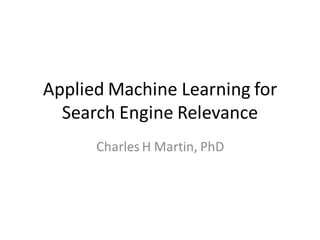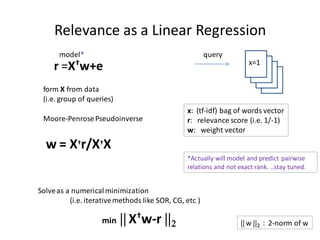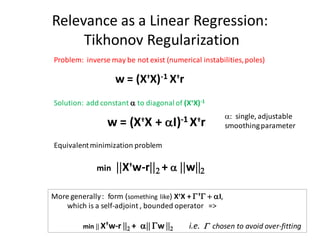Successfully reported this slideshow.

# Applied Machine Learning For Search Engine Relevance×

1 of 32
1 of 32

# Applied Machine Learning For Search Engine Relevance

Application of state-of-the-art machine learning technologies to search engine relevance

Application of state-of-the-art machine learning technologies to search engine relevance

## More Related Content

### Applied Machine Learning For Search Engine Relevance

1. 1. Applied Machine Learning for Search Engine Relevance Charles H Martin, PhD
2. 2. Relevance as a Linear Regression model* query x=1 r =X†w+e form X from data (i.e. group of queries) x: (tf-idf) bag of words vector Moore-Penrose Pseudoinverse r: relevance score (i.e. 1/-1) w: weight vector w = X†r/X†X *Actually will model and predict pairwise relations and not exact rank. ..stay tuned. Solve as a numerical minimization (i.e. iterative methods like SOR, CG, etc )  X†w-r  2 min  w  2 : 2-norm of w
3. 3. Relevance as a Linear Regression: Tikhonov Regularization Problem: inverse may be not exist (numerical instabilities, poles) w = (X†X)-1 X†r Solution: add constant a to diagonal of (X†X)-1 a: single, adjustable aI)-1 X†r w = (X X + † smoothing parameter Equivalent minimization problem X†w-r 2 + a w2 min More generally : form (something like) X†X + G †G + aI, which is a self-adjoint , bounded operator => min  X†w-r 2 + a Gw 2 i.e. G chosen to avoid over-fitting
4. 4. The Representer Theorem Revisited: Kernels and Greens Functions Problem: to estimate a function f(x) from training data (xi) f(x) = S aiR(x, xi) R := Kernel Solution: solve a general minimization problem min Loss[(f(xi), yi)] + aT Ka Kij = R(xi, xj) Equivalent to: given a Linear regularization operator ( G: H->L2(x) ) f(x) = S aiR(x, xi) + S buu (xi) ; u span null space of G min Loss[(f(xi), yi)] + a Gx2 K is an integral operator: (Kf)(y) = R(x,y)f(x)dx where so K is the Green’s Function for (GG) †, or G = (K1/2)† R(x,y) = <y|(GG) †|x> in Dirac Notation: Machine Learning Methods for Estimating Operator Equations (Steinke & Scholkpf 2006)
5. 5. Personalized Relevance Algorithms: eSelf Personality Subspace p q personality traits pages ads Rock-n-roll Cars: 0.4 Sports cars Learned Traits: 0.0 =>0.3 User Hard rock (Likes cars 0.4) Reading (Sports cars 0.3) Present to user (music site) (used sports car ad) Compute personality traits during user visit to web site q values = stored learned “personality traits” Provide relevance rankings (for pages or ads) which include personality traits
6. 6. Personalized Relevance Algorithms: eSelf Personality Subspace p: output nodes q: hidden nodes (observables) (not observed) Individualized Personality Traits u: user segmentation h: history (observed outputs) Web pages, Classified Ads, … L [p,q] = [h, u] model: where L is a square matrix
7. 7. Personalized Search: Effective Regression Problem [p, q](t) = (Leff[q(t-1)]) -1 • [h, u](t) on each time step (t) PLP PLQ p = h PLP p + PLQ q = h => QLP QLQ q u QLP p +QLQ q = 0 Leff p = h Formal solution: p = (Leff [q,u])-1 h Leff = (PLP + PLQ (QLQ)-1 QLP) Adapts on each visit, finding relevant pages p(t) based on the links L, and the learned personality traits (q(t-1)) Regularization of PLP achieved with “Green’s Function / Resolvent Operator” i.e. G †G ~= PLQ (QLQ)-1 QLP Equivalent to Gaussian Process on a Graph, and/or Bayesian Linear Regression
8. 8. Related Dimensional Noise Reductions: Rank (k) Approximations of a Matrix Latent Semantic Analysis (LSA) PDP PDQ (Truncated) Singular Value Decomposition (SVD): Diagonalize the Density operator D = A†A QDP QDQ Retain a subset of (k) eigenvalues/vectors Equivalent relations for SVD (D-X)  2 2 X s.t. min Optimal rank(k) apprx. Decomposition: A = U∑ V† A†A = V (∑† ∑) V† Can generalize to various noise models: i.e. VLSA*, PLSA** ] min E [ qT (D-X)  2 2 VLSA* provides a rank (k) apprx. to any q query: min DKL [P -P(data)] PLSA* provides a rank (k) apprx. of classes (z) P = U∑ V† P(d,w) = P(d|z) P(z)P(w|z) D KL = Kullback–Leibler divergence *Variable Latent Semantic Indexing (Yahoo! Research Labs) **Probabilistic Latent Semantic Indexing (Recommind, Inc) http://www.cs.cornell.edu/people/adg/html/papers/vlsi.pdf http://www.cs.brown.edu/~th/papers/Hofmann-UAI99.pdf
9. 9. Personalized Relevance: Mobile Services and Advertising France Telecom: Last Inch Relevance Engine time location suggest play game send msg play song …
10. 10. KA for Comm Services Comm p Events q Personal Context Services (Sun. mornings) Call [who] Mom (5) SMS [who] Bob (3) MMS [who] Phone company (1) Events Learned Traits: Map to a contextual Suggestions for user On Sunday morning, comm service (Call, SMS, MMS, E-mail) most likely to call Mom • Based on Empirical Bayesian score and Suggestion mapping table, a decision is made to one or more possible Comm services • Based on Business Intelligence (BI) data mining and/or Pattern Recognition algorithms (i.e. supervised or unsupervised learning) , we compute statistical scores indicating who are the most likely people to Call, send an SMS, MMS, or E-Mail.
11. 11. Comm/Call Patterns POD Day of Week LOC calls to different #'s p( |POD ) > p( |SUN); p( |POD) < p( |SUN) p( , P|POD) > 0; p( , )=0
12. 12. Bayesian Score Estimation To estimate p(call|POD) frequency: p(call|POD) = # of times user called someone at that POD Bayesian: p(call|POD) = p(POD|call)p(call) Sq p(POD|q)p(q) where q = call, sms, mms, or email
13. 13. i.e. Bayesian Choice Estimator • We seek to know the probability of a quot;callquot; (choice) at a given POD. • We quot;borrow informationquot; from other PODs, assuming this is less biased, to improve our statistical estimate f( | 1) = 2/5 frequency estimator 1 2 p( | 1) = (2/5)(3/15) . 3 (2/5)(3/15)+(2/5)(3/15)+(1/5)(11/15) 5 days Bayesian choice estimator 3 PODs = 6/23 ~ 1/4 Note: the Bayesian estimate is 3 choices significantly lower because we now expect we might see a at POD 1
14. 14. Incorporating Feedback • It is not enough to simple recognize call patterns in the Event Facts—it is also necessary to incorporate feedback into our suggestions scores • p( c | user, pod, loc, facts, feedback ) = ? Event Facts A: Simply Factorize: p( c | user, pod, facts, feedback ) = p( c | user, pod, facts ) p ( c | user, pod, feedback) Suggestions Evaluate probabilities independently, irrelevant perhaps using different Bayesian models poor good random
15. 15. Personalized Relevance: Empirical Bayesian Models Closed form models: Correct a sample estimate (mean m, variance ) with a i.e: weighted average of sample + complete data set B shrinkage m = Bm + (1-B) m factor user individual segment sample Can rank order mobile services based on estimated likelihood (m , ) play game send msg play song 1 3 1 2
16. 16. Personalized Relevance: Empirical Bayesian Models What is Empirical Bayes modeling? specify Likelihood L(y|) and Prior () distributions estimate the posterior () = L(y|) () L(y|) ()  L(y|) ()d (marginal) Combines Bayesianism and frequentism: Approximates marginal using (or posterior) using point estimate(MLE), Monte Carlo, etc. Estimates marginal using empirical data Uses empirical data to infer prior, plug into likelihood to make predictions Note: Special case of Effective Operator Regression: P space ~ Q space ; PLQ = I ; u  0 Q-space defines prior information
17. 17. Empirical Bayesian Methods: Poisson Gamma Model Likelihood L(y| ) = Poisson distribution ( y e- )/y! Conjugate Prior ( a,b) = Gamma distribution ( a-1 b a e-b a )/ G(a) ;  > 0 posterior (k) L(y|) () = (( y e- )/y!) (( a-1 b a e-b a )/ G(a) ) y+a-1 e-(1+(1/b)) also a Gamma distribution(a’,b’) a’ = y + a ; b’ = (1+1/b)-1 Take MLE estimate of Marginal = mean (m) of the posterior (ab) Obtain a,b from the mean (m = ab) and variance (ab2) of complete data Final Point estimate E(y)= a’b’ for a sample is a weighted average of sample mean y=my and prior mean m E(y) = ( my + a ) (1+1/b)-1 E(y) = (b/1+b) my + (1/1+b) m
18. 18. Linear Personality Matrix suggestions actio n events Linear (or non-linear) Matrix transformation: M s = a Over time, we can estimate the Ma,s = prob( a | s ) and can then solve for the prob( s ) using a computational linear solver: s = M-1a Notice: the personality matrix may or may not mix suggestions across events, can include semantic information i.e. calls i.e. for a given time and location… s1 = call count how many times we suggested a call but the user chose an email instead s2 = sms s3 = mms Obviously we would like M to be diagonal…or as good as possible ! s4 = email Can we devise an algorithm that will learn to give quot;optimalquot; suggestions?
19. 19. Matrices for Pattern Recognition (Statistical Factor Analysis) We can use apply Computational Linear Algebra to remove noise and find patterns in data. Called Factor Analysis by Statisticians, Singular Value Decomposition (SVD) by Engineers. Implemented in Oracle Data Mining (ODM) as Non-Negative Matrix Factorization Week 1 2 3 4 5 … Call on Mon @ pod 1 4. Weekly patterns are Call on Mon @ pod 2 collapsed into the density Call on Mon @ pod 3 Matrix AtA … … They can be detected using spectral analysis Sms on Tue @ pod 1 (i.e. principal eigenvalues) … 2. Count # of times a choice 3. Form weekly choice 1. Enumerate all choices is made each week density Matrix AtA All weekly patterns Pure Noise Similar to Latent (Multinomial) Dirichlet Algorithm (LDA), but much simpler to implement. Suitable when the number (#) of choices is not too large, and patterns are weekly.
20. 20. Search Engine Relevance : Listing on Which 5 items to list at bottom of page ?
21. 21. Statistical Machine Learning: Support Vector Machines (SVM) From Regression to Classification: Maximum Margin Solutions 2 w2 Classification := Find the line that separates the points with the maximum margin w min ½ w2 2 subject to constraints all “above” line all “below” line perhaps within some slack (i.e. min ½ w2 2 + C S I ) constraint specifications: “above” : w.xi –b >= 1 + I “below” : w.xi –b <= 1 + i Simple minimization (regression) becomes a convex optimization (classification)
22. 22. SVM Light: Multivariate Rank Constraints wTx x y Multivariate Classification: x1 - 0.1 -1 x2 +1.2 +1 let Ψ(x,y’) = S y’x be a linear fcn sgn … … … xn -0.7 -1 maps docs to relevance scores (+1/-1) learn weights (w) s.t. max wT Ψ(x,y’) is correct for training set wT Ψ(x,y’) max (within a single slack constraint ) ½ w2 2 +C s.t. min for all y’: wT Ψ(x,y) - wT Ψ(x,y’) >= D(y,y’) -  Ψ(x,y’) is a linear discriminant function: (i.e. sum of ordered pairs S S yij (xi -xj) ) ij D(y,y’) is a multivariate loss function: (i.e. 1- Average Precision(y,y’) )
23. 23. SvmLight Ranking SVMs SVMrank : Ordinal Regression Stnd Classification on pairwise differences S I,j,k min ½ w2 2 + C s.t for all queries qk (later, may not be query specific in SVMstruct) wT Ψ(qk,di) - wT Ψ(qk,dj) >= 1- I,j,k doc pairs di, dj SVMperf : ROC Area, F1 Score, Precision/Recall DROCArea = 1- # swapped pairs SVMmap : Mean Average Precision ( warning: buggy ! ) Enforces a directed ordering 1 2 3 4 5 6 7 8 MAP ROC Area 1 0 0 0 0 1 1 0 8 7 6 5 4 3 2 1 0.56 0.47 1 2 3 4 5 6 7 8 0.51 0.53 A Support Vector Method for Optimizing Average Precision (Joachims et. Al. 2007)
24. 24. Large Scale, Linear SVMs • Solving the Primal – Conjugate Gradient – Joachims: cutting plane algorithm – Nyogi • Handling Large Numbers of Constraints • Cutting Plane Algorithm • Open Source Implementations: – LibSVM – SVMLight
25. 25. Search Engine Relevance : Listing on A ranking SVM consistently improves Shopping.com <click rank> by %12
26. 26. Various Sparse Matrix Problems: Google Page Rank algorithm Ma = a rank a series of web pages by simulating user browsing patterns (a) based on probabilistic model (M) of page links Pattern Recognition, Inference Lp=h estimate unknown probabilities (p) based on historical observations (h) and probability model (L) of links between hidden nodes Quantum Chemistry H =E compute color of dyes, pigments given empirical information on realted molecules and/or solving massive eigenvalue problems
27. 27. Quantum Chemistry: the electronic structure eigenproblem Solve a massive eigenvalue problem (109-1012) H  (, , …) =   (, , …) H nergy Matrix  quantum state eigenvector E ,  , … electrons Methods can have general applicability: Davidson method for dominant eigenvalue / eigenvectors Motivation for Personalization Technology From solution of understanding the conceptual foundations of semi-empirical models (noiseless dimensional reduction)
28. 28. Relations between Quantum Mechanics and Probabilistic Language Models • Quantum States  resemble the states (strings, words, phrases) in probabilistic language models (HMMs, SCFGs), except:  is a sum* of strings of electrons:  (, ) = 0. |  1  2  1  2 | + 0.2 |  2  3  1  2 | + … *Not just a single string! • Energy Matrix H is known exactly, but large. Models of H can be inferred from empirical data to simplify computations. • Energies ~= Log [Probabilities], un-normalized
29. 29. Dimensional Reduction in Quantum Chemistry: where do semi-empirical Hamiltonians come from? Ab initio (from first principles): Solve entire H  (, ) =   (, ) …approximately OR Semi-empirical: Assume (, ) electrons statistically independent:  (, ) = p() q() Treat  -electrons explicitly, ignore  (hidden): PHP p() =  p() much smaller problem Parameterize PHP matrix => Heff with empirical data using a small set of molecules, then apply to others (dyes, pigments)
30. 30. Effective Hamiltonians: Semi-Empirical Pi-Electron Methods Heff [] p() =  p() implicit / hidden PHP PHQ p= E p PHP p + PHQ q = E p => QHP QHQ q q QHP p + QHQ q = E q Heff [] = (PHP – PHQ (E-QHQ)-1 QHP) Final Heff can be solved iteratively (as with eSelf Leff), or perturbatively in various forms Solution is formally exact => Dimensional Reduction / “Renormalization”
31. 31. Graphical Methods Vij = + + +… Decompose Heff into effective interactions between  electrons (Expand (E-QHQ)-1 in an infinite series, remove E dependence) Represent diagrammatically, ~300 diagrams to evaluate Precompile using symbolic manipulation: ~35 MG executable; 8-10 hours to compile run time: 3-4 hours/parameter
32. 32. Effective Hamiltonians: Numerical Calculations -only effective empirical VCC 16 11.5 11-12 (eV) example Compute ab initio empirical parameters : Can test all basic assumptions of semi-empirical theory , “from first principles” Also provides highly accurate eigenvalue spectra Augment commercial packages (i.e. Fujitsu MOPAC) to model spectroscopy of photoactive proteins